# When Are Two Lines Parallel? (3 Methods To Find Out)

Parallel lines are used often in geometry (for proofs) or in algebra (as the result of systems of linear equations).  No matter how you use this important concept, it helps to know when two lines are parallel.

So, when are two lines parallel?  Parallel lines have the same slope but different y-intercepts, which means that the two lines never intersect. That is, there is no point that lies on both lines. Parallel lines are always the same distance apart. A transversal intersecting the two lines will form congruent corresponding angles.

Of course, you might not always have a graph of the two lines available.  In that case, it might be faster (and more accurate) to examine the equations than to graph both lines.

In this article, we’ll talk about when two lines are parallel and how you can tell.  We’ll also look at some examples of parallel lines to make the concepts clear.

Let’s get started.

## When Are Two Lines Parallel?

Two lines are parallel if their graphs never intersect.  There are a few ways to tell when two lines are parallel:

• Check their slopes and y-intercepts: if the two lines have the same slope, but different y-intercepts, then they are parallel.
• Check the distance between them: if two lines always have the same distance between them, then they are parallel.
• Check the angles from a transversal: if a transversal intersecting the two lines forms congruent corresponding angles, then the lines are parallel.

The image below summarizes the 3 possible cases for the solutions for a system of 2 linear equations in 2 variables.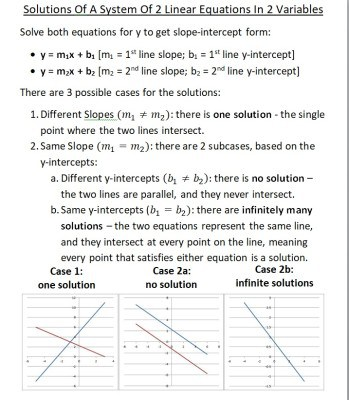We can compare the slopes and y-intercepts of two linear equations in two variables to find out how many solutions there are: 0, 1, or infinitely many.

Let’s take a look at each one in turn.

### How To Tell If Two Lines Are Parallel From Equations

The basic idea here is to look at the slope and y-intercept of each of the two lines.  The four possible cases are as follows:

• Same slope, same y-intercept: the two lines are the same.  In this case, we can say that they intersect everywhere, or that one lies on top of the other.  The two lines come from the same equation.
• Same slope, different y-intercept: the two lines are parallel.  In this case, the two lines never intersect, and they are the same distance apart.
• Different slope, same y-intercept: the two lines intersect at one point: their shared y-intercept.
• Different slope, different y-intercept: the two lines intersect at one point, which is not their shared y-intercept.

The table below summarizes the above cases at a glance.

If the two lines have equations

• y = m1x + b1
• y = m2x + b2

then the slopes are m1, m2 and the y-intercepts are b1, b2.

To show that two lines are parallel, we need:

• m1 = m2 (the two lines have the same slope)
• b1 != b2 (the two lines have different y-intercepts)

Let’s look at some examples.

#### Example 1: Two Lines That Are Parallel

Consider the two lines

• 10x + 2y = 8
• -15x – 3y = 6

We can solve both equation for y (to convert to slope-intercept form).  This will make it easier to compare the slopes and y-intercepts.

For the first equation:

• 10x + 2y = 8
• 2y = -10x + 8  [subtract 10x from both sides]
• y = -5x + 4  [divide by 2 on both sides]

For the second equation:

• -15x – 3y = 6
• –3y = 15x + 6  [add 15x to both sides]
• y = -5x – 2  [divide by -3 on both sides]

Looking at both equations, we can see that they both have a slope of -5.

We can also see that the y-intercepts are different: +4 for the first line, and -2 for the second line.

So, the two lines are parallel, since they have the same slope and different y-intercepts.

You can see the graph of the two lines (which never intersect) pictured below.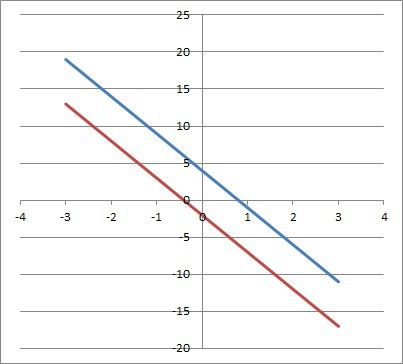The graph of the two parallel lines y = -5x + 4 and y = -5x – 2 shows that they never intersect (same slope of -5, different y-intercepts of 4 and -2).

#### Example 2: Two Lines That Are Not Parallel (Different Slopes)

Consider the two lines

• 8x + 4y = 12
• -20x – 5y = 15

For a linear equation in standard form (Ax + By = C), the slope is given by –A / B.

For the first line, the slope is –A / B = -8 / 4 = -2

For the second line, the slope is –A / B = -(-20) / -5 = 20 / -5 = -4

Since the two lines have different slopes, they cannot be parallel.  They will intersect, as you can see from the graph below.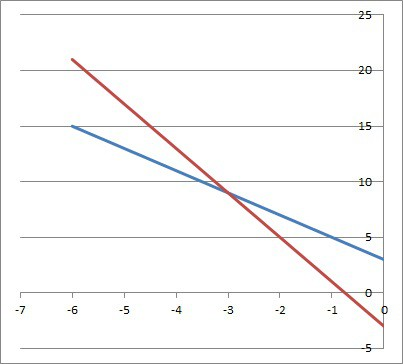The graph of the intersecting lines y = -2x + 3 and y = -4x – 3. They intersect at the point (-3, 9).

#### Example 3: Two Lines That Are Not Parallel (Same Slope, Same Y-Intercept)

Consider the two lines

• 6x + 3y = 9
• 18x + 9y = 27

One shortcut to see that the lines are the same (not parallel) is to note that the second equation is a multiple of the first.

That is, if we multiply the first equation by 3, we get the second equation:

• 6x + 3y = 9  [first equation]
• 3(6x + 3y) = 3(9)  [multiply both sides by 3]
• 18x + 9y = 27  [same as the second equation]

If one linear equation is a constant multiple of another, then the two equations represent the same line on a graph (they both have the same slope and the same y-intercept).

You can see the graph of this line below.

### How To Tell If Two Lines Are Parallel From Their Distance Apart

The basic idea here is to draw a perpendicular line segment to connect one line to the other.  Find the length of the line segment – this is the distance between the two lines.

Then, draw another perpendicular line segment connecting the two lines.  The length of the line segment will be exactly the same as the first one if the lines are parallel.

#### Example: Two Parallel Lines Are The Same Distance Apart

Consider the two lines

• y = x
• y = x – 2

Any perpendicular line to y = x that we draw t will have the opposite reciprocal slope (so, a slope of -1).  The line with a y-intercept of (0, 0) has the equation y = -x.

The line y = -x intersects y = x at the origin (0, 0).

The line y = -x intersects y = x – 2 at the point (1, -1.)

So, the length of the perpendicular line segment is the distance from (0, 0) to (1, -1).  Using the formula for the distance between two points, we get:

• D = √((x2 – x1)2 + (y2 – y1)2)
• D = √((1 – 0)2 + (-1 – 0)2)
• D = √((1)2 + (-1)2)
• D = √(1+ 1)
• D = √2

So, the length of the perpendicular line segment between the two lines is √2.

Now we will draw another perpendicular line with slope -1 and y-intercept 6.  The equation of this line is y = -x + 6.

The line y = -x + 6 intersects y = x at the point (3, 3).

The line y = -x + 6 intersects y = x – 2 at the point (4, 2).

So, the length of the perpendicular line segment is the distance from (3, 3) to (4, 2).  Using the formula for the distance between two points, we get:

• D = √((x2 – x1)2 + (y2 – y1)2)
• D = √((4 – 3)2 + (2 – 3)2)
• D = √((1)2 + (-1)2)
• D = √(1+ 1)
• D = √2

So, the length of the perpendicular line is again √2.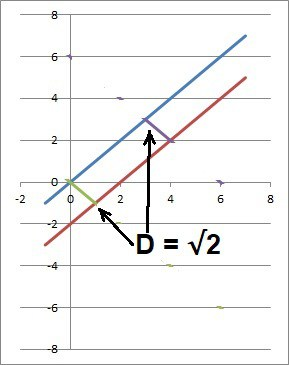The distance between these two parallel lines (y = x and y = x – 2) is always the same: √2.

### How To Tell If Two Lines Are Parallel Using Angles

We can also tell if two angles are parallel by drawing a transversal and examining the corresponding angles.  If they are equal (congruent), then we have two parallel lines.

#### Example 1: Two Parallel Lines Form The Same Angle With A Transversal

Consider the two lines and the transversal in the graph below.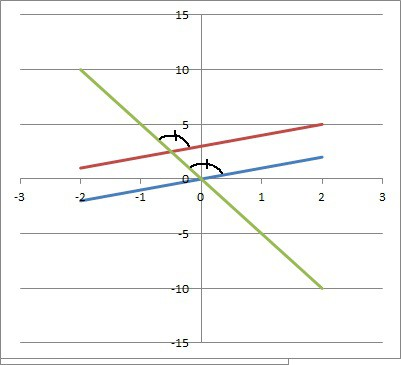The transversal (green line) forms the same corresponding (upper) angle with each of the other two lines (red and blue lines). So, the red and blue lines are parallel.

Since the corresponding angles are equal, this means that the two lines are parallel.

#### Example 2: Two Parallel Lines Form The Same Angle With A Perpendicular

Consider the two lines

• y = x
• y = x – 2

We will draw the transversal y = -x, which passes through both lines: at the origin (0, 0) for the first line and at the point (1, -1) for the second line.

Of course, we know that two lines are perpendicular if the angle formed by them is 90 degrees, or if they have opposite reciprocal slopes.

In this case, the opposite reciprocal of a slope of 1 is -1.

## Conclusion

Now you know a little more about when lines are parallel and the methods you can use to show it.

Remember that when we set two parallel lines equal to find their point of intersection, we get an equation with no solution.

You might also want to read my article on systems of linear equations with no solutions.